## General Chemistry

Definition of acids and bases, identifying them along with the conjugate acid and conjugate base, pH of strong and weak acids and bases, the pH and pOH relationship, Ka and Kb, pKa and Ka correlation, the acid-base properties of salts, identifying the acidity of the salt based on the acid and the base it is prepared from, and calculating the pH of salt solutions. This is what is covered in this summary practice problem set on acids and bases.

The links to corresponding topics are given below:

#### Practice

1.

Identifying Acids and Bases

Write the chemical equation for the ionization reaction and the corresponding Ka equilibrium expression for each acid.

(a) HCN, (b)  H2S, (c)  C5H5NH+, (d) HF

a)

HCN(aq) ⇆ H+(aq) + CN(aq)

${K_{\rm{a}}}\; = \,\frac{{{\rm{[}}{{\rm{H}}^{\rm{ + }}}{\rm{][C}}{{\rm{N}}^{\rm{ – }}}{\rm{]}}}}{{{\rm{[HCN]}}}}$

The ionization can also be shown by the reaction with water:

HCN(aq) + H2O(l) ⇆ H3O+(aq) + CN(aq)

${K_{\rm{a}}}\; = \,\frac{{{\rm{[}}{{\rm{H}}_{\rm{3}}}{{\rm{O}}^{\rm{ + }}}{\rm{][C}}{{\rm{N}}^{\rm{ – }}}{\rm{]}}}}{{{\rm{[HCN]}}}}$

b)

H2S is a diprotic acid and it dissociates in two steps each associated with a separate acid ionization constant.

The dissociation can be shown either with acid or by its reaction with water:

H2S(aq) ⇆ H+(aq) + HS(aq)

${K_{{\rm{a1}}}}\; = \,\frac{{{\rm{[}}{{\rm{H}}^{\rm{ + }}}{\rm{][S}}{{\rm{H}}^{\rm{ – }}}{\rm{]}}}}{{{\rm{[}}{{\rm{H}}_{\rm{2}}}{\rm{S]}}}}$

HS(aq) ⇆ H+(aq) + S2-(aq)

${K_{{\rm{a2}}}}\; = \,\frac{{{\rm{[}}{{\rm{H}}^{\rm{ + }}}{\rm{][}}{{\rm{S}}^{\rm{2}}}^{\rm{ – }}{\rm{]}}}}{{{\rm{[H}}{{\rm{S}}^{\rm{ – }}}{\rm{]}}}}$

The ionization can also be shown by the reaction with water:

H2S(aq) + H2O(l) ⇆ H3O+(aq) + HS(aq)

${K_{{\rm{a1}}}}\; = \,\frac{{{\rm{[}}{{\rm{H}}_{\rm{3}}}{{\rm{O}}^{\rm{ + }}}{\rm{][S}}{{\rm{H}}^{\rm{ – }}}{\rm{]}}}}{{{\rm{[}}{{\rm{H}}_{\rm{2}}}{\rm{S]}}}}$

HS(aq) + H2O(l) ⇆ H3O+(aq) + S2-(aq)

${K_{{\rm{a2}}}}\; = \,\frac{{{\rm{[}}{{\rm{H}}_{\rm{3}}}{{\rm{O}}^{\rm{ + }}}{\rm{][}}{{\rm{S}}^{\rm{2}}}^{\rm{ – }}{\rm{]}}}}{{{\rm{[H}}{{\rm{S}}^{\rm{ – }}}{\rm{]}}}}$

c)

C5H5NH+ is the conjugate acid of C5H5N (pyridine) which is a weak organic base.

C5H5NH+(aq) ⇆ H+(aq) + C5H5N(aq)

${K_{\rm{a}}}\; = \,\frac{{{\rm{[}}{{\rm{H}}^{\rm{ + }}}{\rm{][}}{{\rm{C}}_{\rm{5}}}{{\rm{H}}_{\rm{5}}}{\rm{N]}}}}{{{\rm{[}}{{\rm{C}}_{\rm{5}}}{{\rm{H}}_{\rm{5}}}{\rm{N}}{{\rm{H}}^{\rm{ + }}}{\rm{]}}}}$

The ionization can also be shown by the reaction with water:

C5H5NH+(aq) + H2O(l) ⇆ H3O+(aq) + C5H5N(aq)

${K_{\rm{a}}}\; = \,\frac{{{\rm{[}}{{\rm{H}}_{\rm{3}}}{{\rm{O}}^{\rm{ + }}}{\rm{][}}{{\rm{C}}_{\rm{5}}}{{\rm{H}}_{\rm{5}}}{\rm{N]}}}}{{{\rm{[}}{{\rm{C}}_{\rm{5}}}{{\rm{H}}_{\rm{5}}}{\rm{N}}{{\rm{H}}^{\rm{ + }}}{\rm{]}}}}$

d)

HF(aq) ⇆ H+(aq) + F(aq)

${K_{\rm{a}}}\; = \,\frac{{{\rm{[}}{{\rm{H}}^{\rm{ + }}}{\rm{][}}{{\rm{F}}^{\rm{ – }}}{\rm{]}}}}{{{\rm{[HF]}}}}$

The ionization can also be shown by the reaction with water:

HF(aq) + H2O(l) ⇆ H3O+(aq) + F(aq)

${K_{\rm{a}}}\; = \,\frac{{{\rm{[}}{{\rm{H}}_{\rm{3}}}{{\rm{O}}^{\rm{ + }}}{\rm{][}}{{\rm{F}}^{\rm{ – }}}{\rm{]}}}}{{{\rm{[HF]}}}}$

2.

In each reaction, identify the acid, base, conjugate acid, and the conjugate base according to the Brønsted–Lowry acid-base theory.

(a) H2SO3(aq) + H2O(l) → H3O+(aq) + HSO3(aq)

(b) CH3NH3+(aq) + OH(aq) → CH3NH2(aq) + H2O(l)

(c) H2PO4(aq) + NH3(aq) → HPO42-(aq) + NH4+(aq)

(d) NH3(aq) + H2O(l) → NH4+(aq) + OH(aq)

(e) CH3CH2NH2(aq) + H2O(l) → CH3CH2NH3+(aq) + OH(aq)

(f) HClO3(aq) + H2O(l) → H3O+(aq) + ClO3(aq)

(g) CH3COO2(aq) + HCN(aq) → CH3COOH(aq) + CN(aq)

(h) C5H5N(aq) + H2O(l) → C5H5NH+(aq) + OH(aq)

(i) CO32-(aq) + H2O(l) → HCO3(aq) + OH(aq)

(j) HBr(aq) + H2O(l) → H3O+(aq) + Br(aq)

(k) HClO(aq) + CH3NH2(aq) → CH3NH3+(aq) + ClO(aq)

(l) HCO3(aq) + C5H5NH+(aq) → H2CO3(aq) + C5H5N(aq)

a)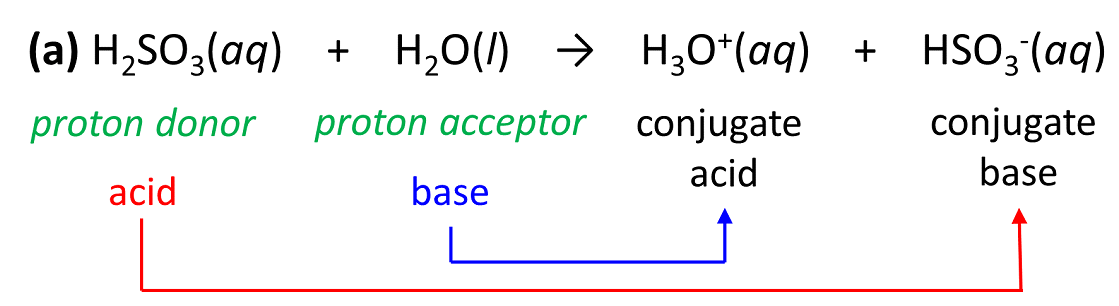b)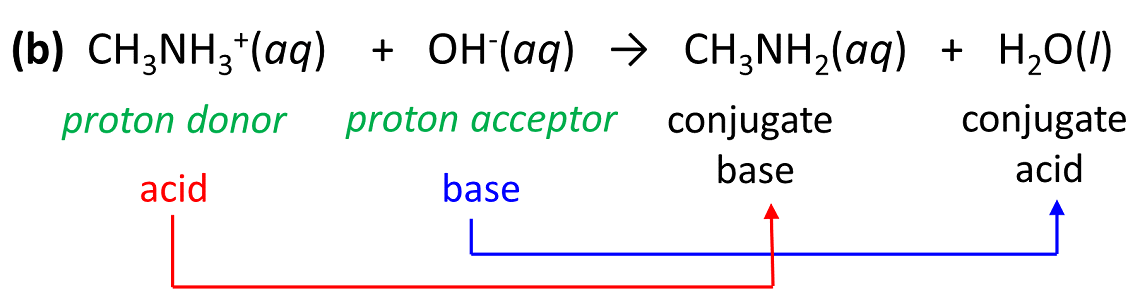c)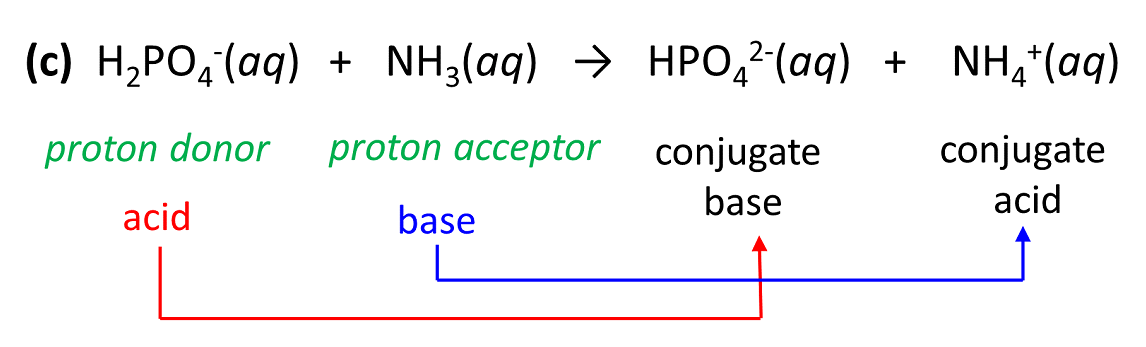d)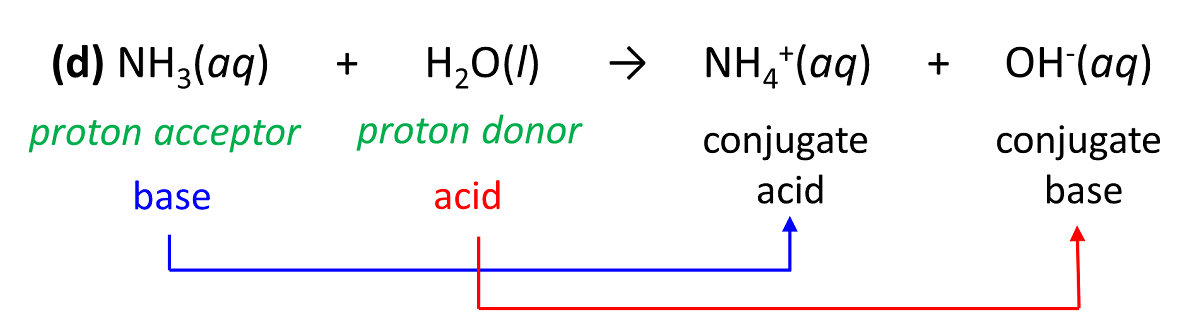e)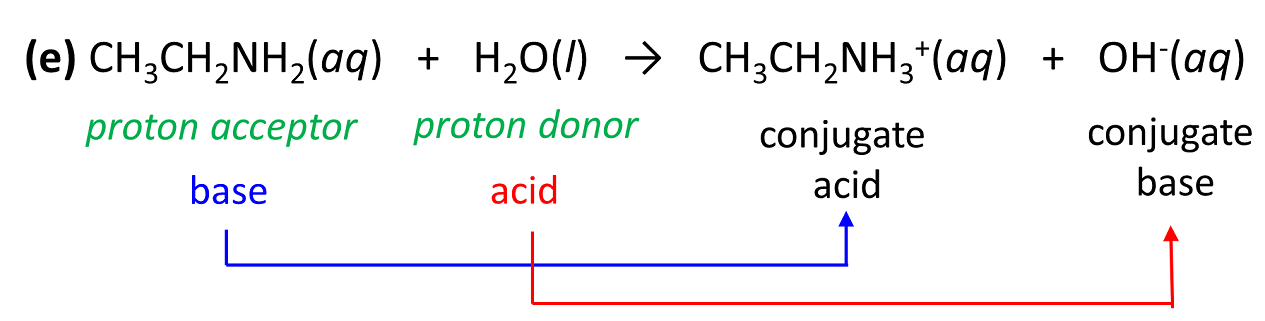f)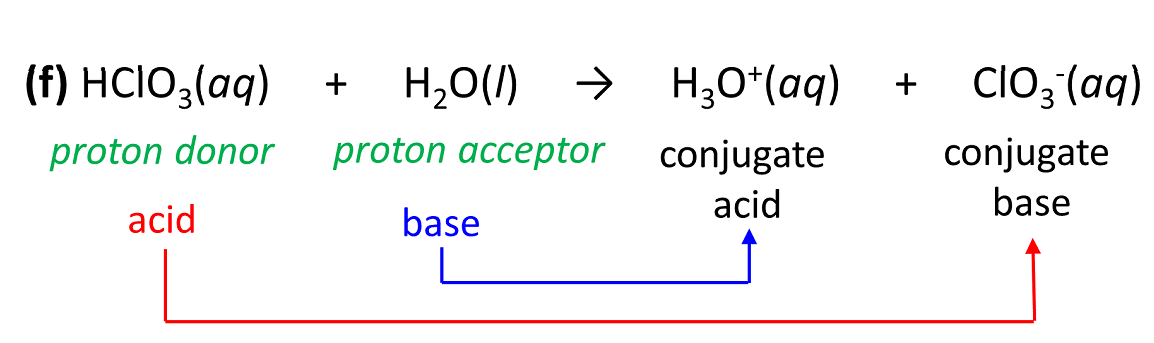g)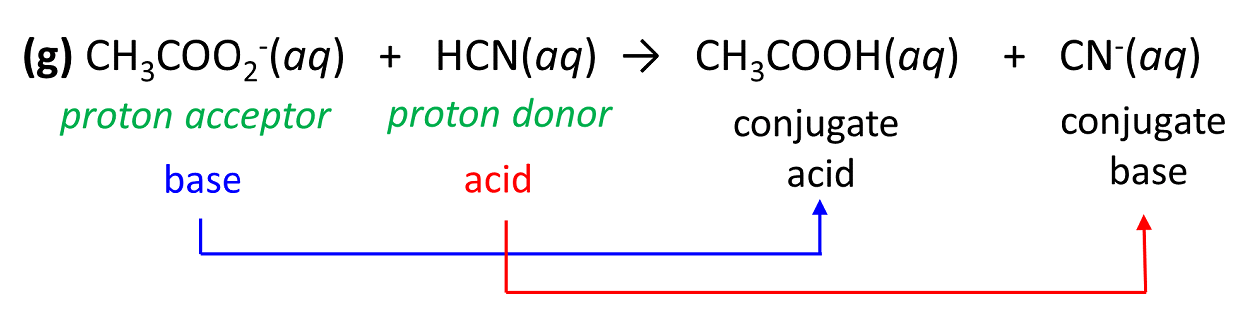h)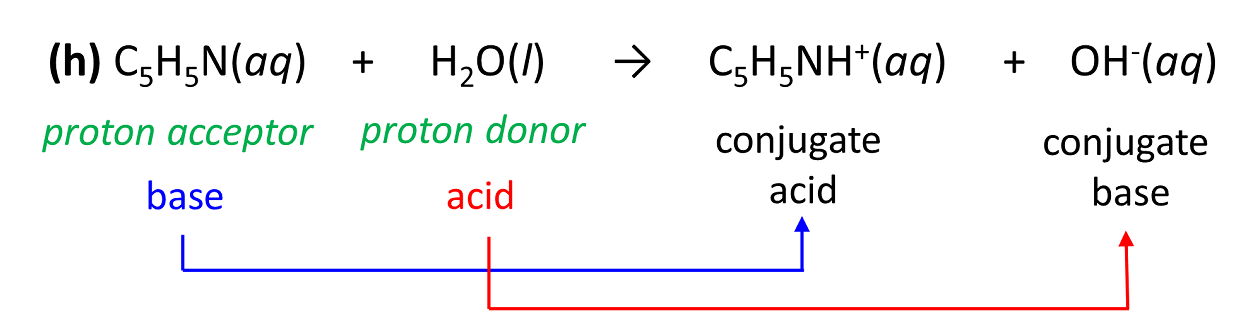i)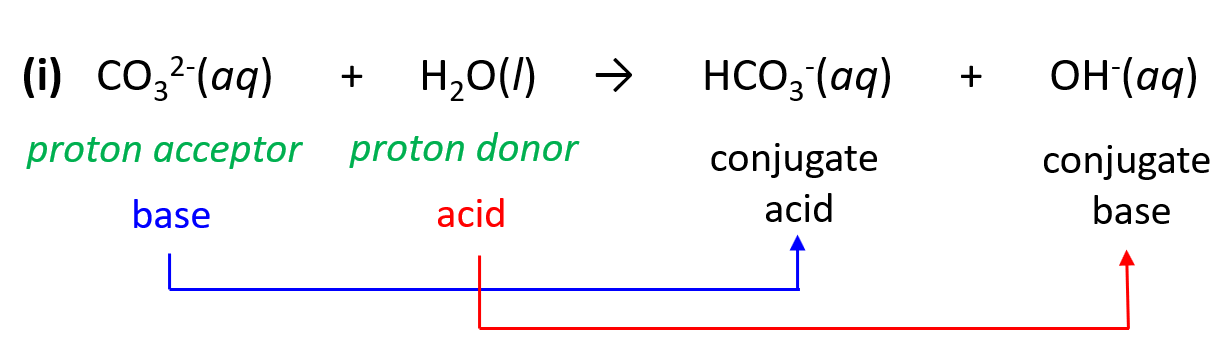j)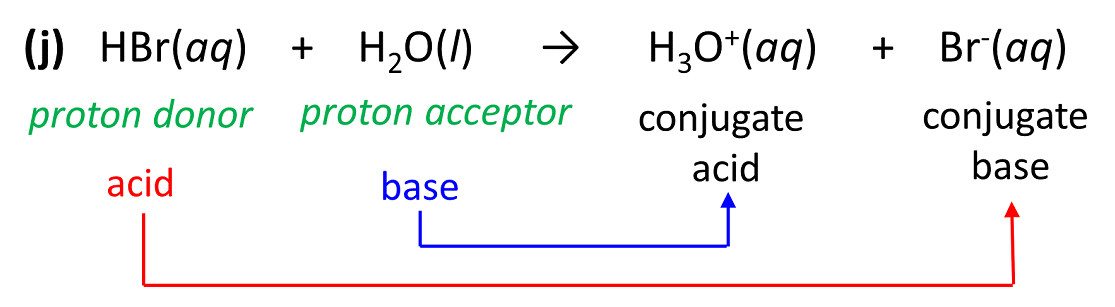k)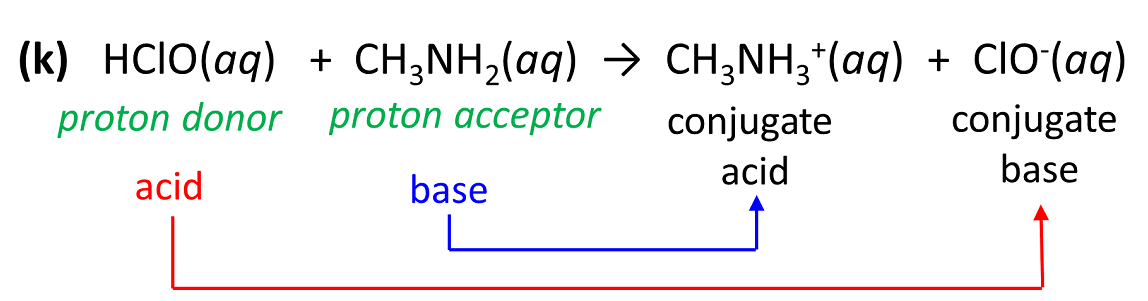l)3.

Acid Strength

Which of the following is the strongest acid: HCl (Ka = 1.3 x 106), CH3CH2NH3+( Ka = 2.5 x 10-11), and H2SO3 (Ka = 5.9 x 10-2)?

HCl

Solution

The acid strength is characterized by their acid-ionization constants. The larger the Ka, the stronger the acid. Notice that it is opposite for the pKa – the smaller the pKa, the stronger the acid.

Among these, HCl is the strongest acid with a Ka of 1.3 x 106. The second strongest would the sulfurous acid, Ka (H2SO3) = 5.9 x 10-2, and the weakest acid here is the protonated ethyl amine, Ka (CH3CH2NH3+) = 2.5 x 10-11.

4.

Which of the following is the weakest acid: HClO3, HCN, or HC2H3O2?

HCN

Solution

The acid strength is characterized by their acid-ionization constants. The larger the Ka, the stronger the acid, and therefore, for a weaker acid, we are looking for a smaller Ka.

HClO3 is a strong acid so it completely dissociates in an aqueous solution.

Ka (HCN) = 6.2 x 10-10

Ka (HC2H3O2) = 1.8 x 10-5

Based on the Ka values, HCN is the weakest acid.

5.

Given the pKa of each acid, determine the stronger conjugate base:

a) H2SO3 (pKa 8.1) vs CH3COOH (pKa 4.75)

b) HClO3 (pKa < 1) vs H3PO4 (pKa12)

c) HCN (pKa 2.1) vs H2SO4(pKa < 1)

d) HNO2 (pKa 3.2) vs HI (pKa < 1)

a)

To answer these types of questions, you need to remember that: 1) Lower pKa indicates a stronger acid, and 2) The stronger the acid the weaker its conjugate base, and vice versa, the weaker the acid, the stronger its conjugate base.

a) H2SO3 (pKa8.1) vs CH3COOH (pKa 4.75)

CH3COOH is a weaker acid, and therefore, its conjugate base CH3COOwill be a stronger base than HSO3.

b)

HClO3 (pKa < 1) vs H3PO4 (pKa 2.12)

H3PO4 is a weaker acid, and therefore, its conjugate base H2PO4will be a stronger base than ClO3.

c)

HCN (pKa 9.21) vs H2SO4(pKa < 1)

HCN is a weaker acid, and therefore, its conjugate base CNwill be a stronger base than HSO4.

d)

HNO2 (pKa 3.32) vs HI (pKa < 1)

HNO2 is a weaker acid, and therefore, its conjugate base NO2will be a stronger base than I.

6.

Autoionization of Water and pH

Arrange the following solutions in the order of increasing acidity (least acidic to most acidic):

(a) pH = 9.8

(b) pH = 1.2

(c) pH = 4.7

(d) pH = 6.4

The lower the pH, the higher the acidity. Therefore,

(a) pH = 9.8 < (d) pH = 6.4 < (c) pH = 4.7 < (b) pH = 1.2

7.

Arrange the following solutions in the order of increasing basicity (least basic to most basic):

(a) pOH = 5.2

(b) pOH = 11.6

(c) pOH = 3.4

(d) pOH = 1.9

The lower the pOH, the higher the basicity. Therefore,

(b) pOH = 11.6 < (a) pOH = 5.2 < (c) pOH = 3.4 < (d) pOH = 1.9

8.

Calculate the pH for each of the following solutions at 25 oC:

(a) [H3O+] = 1.3 x 10-2 M

(b) [H3O+] = 1.6 x 10-3 M

(c) [OH] = 1.8 x 10-3 M

a)

(a) pH = 1.9

b)

(b) pH = 2.8

c)

(c) pH = 11.3

Solution

The pH is defined as the negative logarithm of proton/hydronium ion concentration: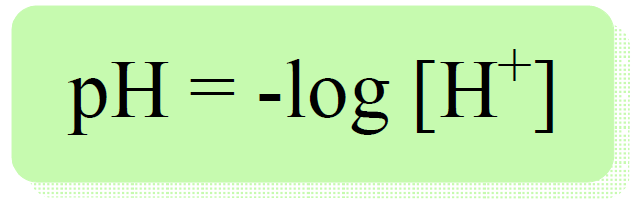(a) pH = -log 1.3 x 10-2 = 1.9

(b) pH = -log 1.6 x 10-3 = 2.8

(c) We can determine the pOH first, and then, using the pH and pOH correlation, calculate the pH.

pOH = -log 1.8 x 10-3 = 2.7

pH + pOH = 14, therefore, pH = 14 – pOH

pH = 14 – 2.7 – 11.3

Alternatively, we can determine the concentration of H+ ions from the relationship [OH] · [H+] = Kw = 10-14.

$\left[ {{{\rm{H}}^{\rm{ + }}}} \right]\;{\rm{ = }}\,\frac{{{K_{\rm{w}}}}}{{\left[ {{\rm{O}}{{\rm{H}}^{\rm{ – }}}} \right]}}\; = \,\frac{{1.0\, \times {{10}^{ – 14}}}}{{1.8\, \times {{10}^{ – 3}}}}\, = \,5.6\, \times \,{10^{ – 12}}$

pH = -log [H+] = -log 5.6 x 10-12 = 11.3

9.

Calculate the [OH] of each of the following solutions at 25 oC. Identify the solution as neutral, acidic, or basic.

a) [H+] = 2.5 x 10-6 M

b) [H+] = 8.3 x 10-4 M

c) [H+] = 4.6 M

d) [H+] = 3.9 x 10-2 M

a)

[OH] = 4.0 x 10-9 M

[OH] < [H+] – acidic

b)

[OH] = 1.2 x 10-11 M

[OH] < [H+] – acidic

c)

[OH] = 2.2 x 10-15 M

[OH] < [H+] – acidic

d)

[OH] = 2.6 x 10-13 M

[OH] < [H+] – acidic

Solution

The [OH] and [H+] can be calculated from one other using their relationship [OH] · [H+] = Kw = 10-14.

${\rm{(a)}}\,\left[ {{\rm{O}}{{\rm{H}}^{\rm{ – }}}} \right]\;{\rm{ = }}\,\frac{{{K_{\rm{w}}}}}{{\left[ {{{\rm{H}}^{\rm{ + }}}} \right]}}\; = \,\frac{{1.0\, \times {{10}^{ – 14}}}}{{2.5\, \times \,{{10}^{ – 6}}}}\, = \,4.0\, \times \,{10^{ – 9}}$

${\rm{(b)}}\,\left[ {{\rm{O}}{{\rm{H}}^{\rm{ – }}}} \right]\;{\rm{ = }}\,\frac{{{K_{\rm{w}}}}}{{\left[ {{{\rm{H}}^{\rm{ + }}}} \right]}}\; = \,\frac{{1.0\, \times {{10}^{ – 14}}}}{{8.3\, \times \,{{10}^{ – 4}}}}\, = \,1.2\, \times \,{10^{ – 11}}$

${\rm{(c)}}\,\left[ {{\rm{O}}{{\rm{H}}^{\rm{ – }}}} \right]\;{\rm{ = }}\,\frac{{{K_{\rm{w}}}}}{{\left[ {{{\rm{H}}^{\rm{ + }}}} \right]}}\; = \,\frac{{1.0\, \times {{10}^{ – 14}}}}{{4.6}}\, = \,2.2\, \times \,{10^{ – 15}}$

${\rm{(d)}}\,\left[ {{\rm{O}}{{\rm{H}}^{\rm{ – }}}} \right]\;{\rm{ = }}\,\frac{{{K_{\rm{w}}}}}{{\left[ {{{\rm{H}}^{\rm{ + }}}} \right]}}\; = \,\frac{{1.0\, \times {{10}^{ – 14}}}}{{3.9\, \times \,{{10}^{ – 2}}}}\, = \,2.6\, \times \,{10^{ – 13}}$

10.

Given the pH values, calculate [H3O+] and [OH] for each solution at 25 oC. Identify each solution as neutral, acidic, or basic.

a) pH = 7.20

b) pH = 15.3

c) pH = 4.60

a)

pH > 7 – basic

[H3O+] = 6.31 x 10-8

[OH] = 1.58 x 10-7

b)

pH >> 7 – strongly basic

[H3O+] = 5.01 x 10-16

[OH] = 20.

c)

pH < 7 – acidic

[H3O+] = 2.51 x 10-5

[OH] = 3.98 x 10-10

Solution

The [H3O+] can be determined by the antilog of the pH. The [OH], in turn, is calculated from the [H3O+]  and [OH] relationship.

a) pH = 7.20

Step 1. Determine the [H3O+]

[H3O+] = 10-pH = 10-7.20 = 6.31 x 10-8

Step 2. Determine the [OH] using their relationship [OH] · [H+] = Kw = 10-14.

$\left[ {{\rm{O}}{{\rm{H}}^{\rm{ – }}}} \right]\;{\rm{ = }}\,\frac{{{K_{\rm{w}}}}}{{\left[ {{{\rm{H}}^{\rm{ + }}}} \right]}}\; = \,\frac{{1.0\, \times {{10}^{ – 14}}}}{{6.31\, \times \,{{10}^{ – 8}}}}\, = \,1.58\, \times \,{10^{ – 7}}$

The solution is basic because [H3O+] < [OH] the pH > 7.

b) pH = 15.3

Step 1. Determine the [H3O+]

[H3O+] = 10-pH = 10-15.3 = 5.01 x 10-16

Step 2. Determine the [OH] using their relationship [OH] · [H+] = Kw = 10-14.

$\left[ {{\rm{O}}{{\rm{H}}^{\rm{ – }}}} \right]\;{\rm{ = }}\,\frac{{{K_{\rm{w}}}}}{{\left[ {{{\rm{H}}^{\rm{ + }}}} \right]}}\; = \,\frac{{1.0\, \times {{10}^{ – 14}}}}{{5.01\, \times \,{{10}^{ – 16}}}}\, = \,20.$

The solution is basic because [H3O+] < [OH] the pH > 7.

c) pH = 4.60

Step 1. Determine the [H3O+]

[H3O+] = 10-pH = 10-4.60 = 2.51 x 10-5

Step 2. Determine the [OH] using their relationship [OH] · [H+] = Kw = 10-14.

$\left[ {{\rm{O}}{{\rm{H}}^{\rm{ – }}}} \right]\;{\rm{ = }}\,\frac{{{K_{\rm{w}}}}}{{\left[ {{{\rm{H}}^{\rm{ + }}}} \right]}}\; = \,\frac{{1.0\, \times {{10}^{ – 14}}}}{{2.51\, \times \,{{10}^{ – 5}}}}\, = \,3.98\, \times \,{10^{ – 10}}$

The solution is acidic because [H3O+] > [OH] the pH < 7.

11.

The pH of Strong Acids and Bases

Calculate the pH for each of the following solutions:

(a) 0.15 M HCl

(b) 0.60 M HClO4

(c) 1.4 M KOH

a)

pH = 0.82

b)

pH = 0.22

c)

pH = 14.15

Solution

Strong acids and bases completely dissociate in aqueous solutions, and therefore, the H+ concentration is going to be the same as the concentration of the monoprotic acid.

(a) 0.15 M HCl

pH = -log [H+] = -log 0.15 = 0.82

(b) 0.60 M HClO4

pH = -log [H+] = -log 0.60 = 0.22

(c) 1.4 M KOH

Strong acids and bases completely dissociate in aqueous solutions, and therefore, the [OH] is going to be the same as the [KOH].

We can determine the pOH first, and then, using the pH and pOH correlation, calculate the pH.

pOH = -log 1.4 = -0.15

pH + pOH = 14, therefore, pH = 14 – pOH

pH = 14 – (-0.15) = 14.15

12.

Calculate the pH of each of the following solutions:

(a) 0.0025 M HI, (b) 0.84 M NaOH

a)

pH = 2.6

b)

pH = 13.924

Solution

(a) Strong acids and bases completely dissociate in aqueous solutions, and therefore, the H+ concentration is going to be the same as the concentration of the monoprotic acid.

pH = -log [H+] = -log 0.0025 = 2.6

(b) 0.84 M NaOH

Strong acids and bases completely dissociate in aqueous solutions, and therefore, the [OH] is going to be the same as the [NaOH].

We can determine the pOH first, and then, using the pH and pOH correlation, calculate the pH.

pOH = -log 0.84 = 0.076

pH + pOH = 14, therefore, pH = 14 – pOH

pH = 14 – 0.076 = 13.924

13.

Calculate the pH of the solution prepared by dissolving 24.0 g of HCl in 662 mL of water.

pH = 2.61 x 10-3

Solution

We need to determine the concentration of H+ ions which is equal to the concentration of HCl because it is a strong acid completely dissociated in water.

So, the first step is to calculate the moles of HCl and determine the molarity.

${\rm{n}}\,{\rm{(HCl)}}\,{\rm{ = }}\,\frac{{\rm{m}}}{{\rm{M}}}\,{\rm{ = }}\,\frac{{{\rm{24}}{\rm{.0}}\,{\rm{g}}}}{{{\rm{36}}{\rm{.5}}\,{\rm{g/mol}}}}\,{\rm{ = }}\,{\rm{0}}{\rm{.658}}\,{\rm{mol}}$

${\rm{M}}\,{\rm{(HCl)}}\,{\rm{ = }}\,\frac{{\rm{n}}}{{\rm{M}}}\,{\rm{ = }}\,\frac{{{\rm{0}}{\rm{.658}}\,{\rm{mol}}}}{{{\rm{0}}{\rm{.662 L}}}}\,{\rm{ = }}\,{\rm{0}}{\rm{.994}}\,{\rm{mol/L}}$

pH = – log [H+] = – log 0.994 = 0.00261 = 2.61 x 10-3

14.

How many grams of KOH is needed to prepare a 680.0 mL solution with a pH of 9.80?

2.41 x 10-3 g

Solution

We need to determine the concentration of OH ions which is equal to the concentration of KOH because it is a strong base and completely dissociates in water.

So, we need to calculate the moles of KOH from the pH. For this, let’s first determine the [H+] from the pH and then, the [OH] using the relationship [OH] · [H+] = Kw = 10-14.

Step 1. Determine the concentration of H+ ions:

pH = – log [H+], [H+] = 10-pH

[H+] = 10-9.80 = 1.58 x 10-10

Step 2. Determine the [OH]

$\left[ {{\rm{O}}{{\rm{H}}^{\rm{ – }}}} \right]\;{\rm{ = }}\,\frac{{{K_{\rm{w}}}}}{{\left[ {{{\rm{H}}^{\rm{ + }}}} \right]}}\; = \,\frac{{1.0\, \times {{10}^{ – 14}}}}{{1.58\, \times \,{{10}^{ – 10}}}}\, = \,6.33\, \times \,{10^{ – 5}}$

This is the concentration of OH ions which is equal to the concentration of KOH.

At this point, we know the concentration and the volume of the KOH solution, and to find the mass of KOH, we first calculate its moles from the molarity:

Step 3. Determine the moles of KOH:

${\rm{n }}\left( {{\rm{KOH}}} \right){\rm{ = MV = 6}}{\rm{.33}}\; \times \,{\rm{1}}{{\rm{0}}^{{\rm{ – 5}}}}\,\frac{{{\rm{mol}}}}{{\cancel{{\rm{L}}}}}\;{\rm{ \times }}\,{\rm{0}}{\rm{.6800}}\,\cancel{{\rm{L}}}{\rm{ = }}\,4.30\, \times \,{\rm{1}}{{\rm{0}}^{{\rm{ – 5}}}}\,{\rm{mol}}$

Step 4. Convert the moles to mass:

m (KOH) = 4.30 x 10-5 mol x 56.1 g/mol = 2.41 x 10-3 g

15.

Calculate the pH of the solution prepared by diluting 40.0 mL of 3.00 HNO3 with 210.0 mL of water.

pH = 0.319

Solution

The first step is to determine the new concentration of HNO3 using the dilution formula. So, let’s label the M and V for the stock solution and the new solution:

M1 = 3.00

V1 = 40.0 mL

V2 = 40.0 + 210.0 = 250. mL

M2 = ?

M1V1 = M2V2

${{\rm{M}}_{\rm{2}}}\,{\rm{ = }}\,\frac{{{{\rm{M}}_{\rm{1}}}{{\rm{V}}_{\rm{1}}}}}{{{{\rm{V}}_{\rm{2}}}}}$

${{\rm{M}}_{\rm{2}}}\,{\rm{ = }}\,\frac{{{\rm{3}}{\rm{.00}}\,M\, \times \,{\rm{40}}{\rm{.0}}\,{\rm{mL}}}}{{{\rm{250}}{\rm{.}}\,{\rm{mL}}}}\; = \,0.48\;M$

This is the concentration of HNO3, and because it is a strong acid, the [H+] is also 0.480 M.

Therefore, the pH is:

pH = – log [H+] = – log 0.480 = 0.319

16.

The pH of Weak Acids

Calculate the pH of a 0.45 M solution of HCN. Ka (HCN) = 4.9 x 10-10

pH = 4.8

Solution

First, let’s write the dissociation equation of HCN:

HCN ⇆ H+ + CN

Initially, the concentration of HCN is 0.45 M, and we can assign M as the concentration of HCN that dissociate (change in the ICE table). The equilibrium concentration of HCN is going to be decreased by x mol/l, so it is (0.45 -x) M.

The initial concentration of H+ and CN ions are 0, but at equilibrium, they are going to be x mol/l because that is how much the HCN dissociates.

So, let’s set up an ICE table with these values:

HCN ⇆ H+ + CN

Next, we set up the equation based on the Ka:

${K_{\rm{a}}}\; = \,\frac{{{\rm{[}}{{\rm{H}}^{\rm{ + }}}{\rm{][C}}{{\rm{N}}^{\rm{ – }}}{\rm{]}}}}{{{\rm{[HCN]}}}}\; = \;\frac{{{{\rm{x}}^{\rm{2}}}}}{{{\rm{0}}{\rm{.45}}\;{\rm{ – }}\;{\rm{x}}}}\; = \;{\rm{4}}{\rm{.9 }} \times {\rm{ 1}}{{\rm{0}}^{{\rm{ – 10}}}}$

Now, we make an approximation that 0.45 – x ≈ 045 because the dissociation constant is very small and by doing so, we get a simplified equation:

$\;\frac{{{{\rm{x}}^{\rm{2}}}}}{{{\rm{0}}{\rm{.45}}\;}}\; = \;{\rm{4}}{\rm{.9 }} \times {\rm{ 1}}{{\rm{0}}^{{\rm{ – 10}}}}$

Therefore,

x = 1.4849 x 10-5

Make sure the approximation is valid, and for this, x must be less than 5% of HCN initial concentration (less than 5% of the acid dissociates). 1.4849 x 10-5/0.45 = 0.0033 %, so the approximation is valid.

So, x = 4.1 x 10-5 , and this is the [H+at equilibrium, therefore,

pH = -log 1.4849 x 10-5 = 4.8

The answer is reasonable because we have an acidic solution and the pH < 7.

17.

Calculate the pH of a 0.74 M solution of acetic acid. Ka (CH3CO2H) = 1.7 x 10-5

pH = 2.5

Solution

First, let’s write the dissociation equation of acetic acid:

CH3CO2H ⇆ H+ + CH3CO2

Initially, the concentration of CH3CO2H is 0.74 M, and we can assign M as the concentration of CH3CO2H that dissociates (change in the ICE table). The equilibrium concentration of CH3CO2H is going to be decreased by x mol/l, so it is (0.74 -x) M.

The initial concentration of H+ and CH3CO2 ions are 0, but at equilibrium, they are going to be x mol/l because that is how much the CH3CO2H dissociates.

So, let’s set up an ICE table with these values:

CH3CO2H ⇆ H+ + CH3CO2

Next, we set up the equation based on the Ka:

${K_{\rm{a}}}\;{\rm{ = }}\,\frac{{{\rm{[}}{{\rm{H}}^{\rm{ + }}}{\rm{][C}}{{\rm{H}}_{\rm{3}}}{\rm{C}}{{\rm{O}}_{\rm{2}}}^{\rm{ – }}{\rm{]}}}}{{{\rm{[C}}{{\rm{H}}_{\rm{3}}}{\rm{C}}{{\rm{O}}_{\rm{2}}}{\rm{H]}}}}\;{\rm{ = }}\;\frac{{{{\rm{x}}^{\rm{2}}}}}{{{\rm{0}}{\rm{.74}}\;{\rm{ – }}\;{\rm{x}}}}\;{\rm{ = }}\;{\rm{1}}{\rm{.7 \times 1}}{{\rm{0}}^{{\rm{ – 5}}}}$

Now, we make an approximation that 0.74 – x ≈ 0.74 to get a simplified equation:

$\;\frac{{{{\rm{x}}^{\rm{2}}}}}{{{\rm{0}}{\rm{.74}}\;}}\; = \;{\rm{1}}{\rm{.7 }} \times {\rm{ 1}}{{\rm{0}}^{{\rm{ – 5}}}}$

Therefore,

x = 3.546 x 10-3

Make sure the approximation is valid, and for this, x must be less than 5% of CH3CO2H initial concentration (less than 5% of the acid dissociates). 3.546 x 10-3/0.74 = 0.48 % – the approximation is valid.

So, x = 3.546 x 10-3, and this is the [H+at equilibrium, therefore,

pH = -log 3.546 x 10-3= 2.45 = 2.5

The answer is reasonable because we have an acidic solution and the pH < 7.

18.

0.86 g benzoic acid (C6H5CO2H, Ka = 6.4 x 10-5) was dissolved in enough water to make 1.0 L of solution. Calculate the pH and concentration of all species present in the solution.

pH = 3.2

Solution

The first part here is going to be determining the initial concentration of benzoic acid by converting the mass to moles and dividing it by the volume of the solution.

${\rm{n}}\;{\rm{(}}{{\rm{C}}_{\rm{6}}}{{\rm{H}}_{\rm{5}}}{\rm{C}}{{\rm{O}}_{\rm{2}}}{\rm{H)}}\,{\rm{ = }}\,{\rm{0}}{\rm{.86}}\,\cancel{{\rm{g}}}\,{\rm{ \times }}\,\frac{{{\rm{1}}\;{\rm{mol}}}}{{{\rm{122}}{\rm{.1}}\,\cancel{{\rm{g}}}}}\;{\rm{ = }}\;{\rm{7}}{\rm{.0}}\,{\rm{ \times }}\,{\rm{1}}{{\rm{0}}^{{\rm{ – 3}}}}\,{\rm{mol}}$

Because the volume of the solution is 1.0 L, the molarity is equal to the moles of the acid.

Next, we write the dissociation equation of benzoic acid:

C6H5CO2H ⇆ H+ + C6H5CO2

Initially, the concentration of C6H5CO2H is 7.0 x 10-3 M, and we can assign M as the concentration of CH3CO2H that dissociates (change in the ICE table). The equilibrium concentration of C6H5CO2H is going to be decreased by x mol/l, so it is (7.0 x 10-3 – x) M.

The initial concentration of H+ and C6H5CO2 ions are 0, but at equilibrium, they are going to be x mol/l because that is how much the C6H5CO2H dissociates.

So, let’s set up an ICE table with these values:

C6H5CO2H ⇆ H+ + C6H5CO2

 [C6H5CO2H] [H+] [C6H5CO2–] Initial 7.0 x 10-3 0 0 Change -x +x +x Equil 7.0 x 10-3 – x x x

Next, we set up the equation based on the Ka:

${K_{\rm{a}}}\;{\rm{ = }}\,\frac{{{\rm{[}}{{\rm{H}}^{\rm{ + }}}{\rm{][}}{{\rm{C}}_{\rm{6}}}{{\rm{H}}_{\rm{5}}}{\rm{C}}{{\rm{O}}_{\rm{2}}}^{\rm{ – }}{\rm{]}}}}{{{\rm{[}}{{\rm{C}}_{\rm{6}}}{{\rm{H}}_{\rm{5}}}{\rm{C}}{{\rm{O}}_{\rm{2}}}{\rm{H]}}}}\;{\rm{ = }}\;\frac{{{{\rm{x}}^{\rm{2}}}}}{{{\rm{7}}{\rm{.0 x 1}}{{\rm{0}}^{{\rm{ – 3}}}}\;{\rm{ – }}\;{\rm{x}}}}\;{\rm{ = }}\;{\rm{6}}{\rm{.4 \times 1}}{{\rm{0}}^{{\rm{ – 5}}}}$

Notice that the initial concentration is very low, and the approximation is likely going to be invalid, but to make sure, let’s go ahead and test it.

${K_{\rm{a}}}\;{\rm{ = }}\,\frac{{{{\rm{x}}^{\rm{2}}}}}{{{\rm{7}}{\rm{.0 x 1}}{{\rm{0}}^{{\rm{ – 3}}}}}}\;{\rm{ = }}\;{\rm{6}}{\rm{.4 \times 1}}{{\rm{0}}^{{\rm{ – 5}}}}$

Therefore,

x = 6.69 x 10-4

The percent ionization is 6.69 x 10-4/7.0 x 10-3 = 9.56 %, so the approximation is not valid.

Therefore, we must solve the quadratic equation from the Ka expression.

$\frac{{{{\rm{x}}^{\rm{2}}}}}{{{\rm{7}}{\rm{.0 x 1}}{{\rm{0}}^{{\rm{ – 3}}}}\;{\rm{ – }}\;{\rm{x}}}}\;{\rm{ = }}\;{\rm{6}}{\rm{.4 \times 1}}{{\rm{0}}^{{\rm{ – 5}}}}$

x2 = -6.4 x 10-5x + 4.48 x 10-7

Rearrange to a form of standard quadratic equation:

x2 + 6.4 x 10-5x – 4.48 x 10-7 = 0

${\rm{x}}\;{\rm{ = }}\;\frac{{ – b \pm \sqrt {{b^2} – 4ac} }}{{2a}}$

a = 1, b = 6.4 x 10-5, c = -4.48 x 10-7

Solving this, we find that:

x = 6.3 x 10-4

So, x = 6.3 x 10-4, and this is the [H+] and [C6H5CO2] at equilibrium. The concentration of the acid at equilibrium is the difference between the initial concentration and the change:

[C6H5CO2H] = 7.0 x 10-3 – 6.3 x 10-4 = 6.37 x 10-3 M

The pH is:

pH = -log 6.3 x 10-4= 3.2

Now, let’s see how different the pH would have been if we kept the approximation. The concentration found by the approximation was 6.69 x 10-4, so the pH is:

pH = -log 6.69 x 10-4= 3.17

The difference is insignificant and if we round off the numbers, we get the same answer. However, it is not every time that the answers will be so close, and it’s a good idea to follow the accepted guidelines for the approximation which is the ionization should be less than 5% of the initial concentration of the acid.

To summarize, the concentrations at equilibrium and the pH are going to be:

[C6H5CO2H] = 6.37 x 10-3 M

[C6H5CO2] = 6.3 x 10-4 M

[H+] = 6.3 x 10-4 M

pH = 3.2

19.

Calculate the pH of a 2.0 M solution of hydrofluoric acid, HF if Ka (HF) = 6.6 x 10-4

pH = 1.4

Solution

First, let’s write the dissociation equation of acetic acid:

HF ⇆ H+ + F

Initially, the concentration of HF is 2.0 M, and we can assign M as the concentration of HF that dissociate (change in the ICE table). The equilibrium concentration of HF is going to be decreased by x mol/l, so it is (2.0 – x) M.

The initial concentration of H+ and F ions are 0, but at equilibrium, they are going to be x mol/l because that is how much the HF dissociates.

So, let’s set up an ICE table with these values:

HF ⇆ H+ + F

 [HF] [H+] [F–] Initial 2.0 0 0 Change -x +x +x Equil 2.0 – x x x

Next, we set up the equation based on the Ka:

${K_{\rm{a}}}\;{\rm{ = }}\,\frac{{{\rm{[}}{{\rm{H}}^{\rm{ + }}}{\rm{][}}{{\rm{F}}^{\rm{ – }}}{\rm{]}}}}{{{\rm{[HF]}}}}\;{\rm{ = }}\;\frac{{{{\rm{x}}^{\rm{2}}}}}{{{\rm{2}}{\rm{.0}}\;{\rm{ – }}\;{\rm{x}}}}\;{\rm{ = }}\;{\rm{6}}{\rm{.6 \times 1}}{{\rm{0}}^{{\rm{ – 4}}}}$

Now, we make an approximation that 2.0 – x ≈ 2.0 because, the dissociation constant is very small and by doing so, we get a simplified equation:

$\;\frac{{{{\rm{x}}^{\rm{2}}}}}{{2.0\;}}\; = \;{\rm{6}}{\rm{.6 }} \times {\rm{ 1}}{{\rm{0}}^{{\rm{ – 4}}}}$

Therefore,

x = 0.0363

Make sure the approximation is valid, and for this, x must be less than 5% of HF initial concentration (less than 5% of the acid dissociates). 0.0363/2.0 = 1.82 % – the approximation is valid.

So, x = 0.0363, and this is the [H+]at equilibrium, therefore,

pH = -log 0.0363= 1.4

The answer is reasonable because we have an acidic solution and the pH < 7.

20.

What is the acid ionization constant (Ka) of a weak acid (HA) if its 0.246 M solution has a pH of 2.68?

1.81 x 10-5 M

Solution

First, let’s write the dissociation equation of HA:

HA ⇆ H+ + A

The Ka expression is:

${K_{\rm{a}}}\;{\rm{ = }}\,\frac{{{\rm{[}}{{\rm{H}}^{\rm{ + }}}{\rm{][}}{{\rm{A}}^{\rm{ – }}}{\rm{]}}}}{{{\rm{[HA]}}}}\;$

Now, if we determine the [H+] from the pH, we will also know the [A], and how much of the acid had ionized because the dissociation reaction is a 1:1 ratio.

pH = – log [H+], [H+] = 10-pH

[H+] = 10-2.68 = 0.0021

This is the concentration of the acid that is ionized, therefore, the equilibrium concentration of the acid is:

[HA]eq = [HA]initial – [HA]ionized = 0.246 – 0.0021 = 0.2439

And now that we have all the equilibrium concentrations, we can plug them in the Ka expression and calculate its value:

${K_{\rm{a}}}\;{\rm{ = }}\,\frac{{\left( {0.0021} \right)\left( {0.0021} \right)}}{{{\rm{0}}{\rm{.2439}}}}\; = \;1.81\, \times \,{10^{ – 5\;}}$

21.

A solution of nitrous acid (HNO2, Ka = 7.2 x 10-4) has a pH of 3.1. What was the initial concentration of nitrous acid in the solution?

1.67 x 10-3 M

Solution

Like in the previous example, we can determine the [H+] from the pH, which will also indicate how much of the acid had ionized because the dissociation reaction occurs in a 1:1 ratio.

pH = – log [H+], [H+] = 10-pH

[H+] = 10-3.1 = 7.94 x 10-4

This is the concentration of the acid that ionized, therefore, if we assign x M for the initial concentration of the acid, the equilibrium concentration will be:

[HNO2]eq = [HNO2]initial – [HNO2]ionized = x – 7.94 x 10-4

Now we can set up an ICE table and solve for x:

HNO2 ⇆ H+ + NO2

 [HNO2] [H+] [NO2–] Initial x 0 0 Change -7.94 x 10-4 +7.94 x 10-4 +7.94 x 10-4 Equil x-7.94 x 10-4 7.94 x 10-4 7.94 x 10-4

The Ka expression will be the basis of the equation to determine the x.

${K_{\rm{a}}}\;{\rm{ = }}\,\frac{{{\rm{[}}{{\rm{H}}^{\rm{ + }}}{\rm{][N}}{{\rm{O}}_{\rm{2}}}^{\rm{ – }}{\rm{]}}}}{{{\rm{[HN}}{{\rm{O}}_{\rm{2}}}{\rm{]}}}}\;$

${K_{\rm{a}}}\;{\rm{ = }}\,\frac{{\left( {7.94\, \times \,{{10}^{ – 4}}} \right)\left( {7.94\, \times \,{{10}^{ – 4}}} \right)}}{{{\rm{x}}\; – \,7.94\, \times \,{{10}^{ – 4}}}}\; = \;7.2\, \times \,{10^{ – 4\;}}$

6.305 x 10-7 = 7.2 x 10-4x – 5.717 x 10-7

x = 1.67 x 10-3

And this is the initial concentration of the acid.

22.

Calculate the pH of a 0.40 M H2S solution given that Ka1 = 1.0 x 10-7; Ka2 = 1.0 x 10-19.

pH = 3.7

Solution

H2S is a diprotic acid, and because the acid ionization constant for the second step is very small, we can ignore the H+ contribution from the second step.

H2S(aq) ⇆ H+(aq) + HS(aq) Ka1 = 1.0 x 10-7

HS(aq) ⇆ H+(aq) + S2-(aq) Ka2 = 1.0 x 10-19 (ignore)

Initially, the concentration of H2S is 0.40 M, and we can assign M as the concentration of H2S that dissociate (change in the ICE table). The equilibrium concentration of H2S is going to be decreased by x mol/l, so it is (0.40 – x) M.

The initial concentration of H+ and HS ions is 0, but at equilibrium, they are going be x mol/l because that is how much the HS dissociates.

So, let’s set up an ICE table with these values:

H2S(aq) ⇆ H+(aq) + HS(aq)

 [H2S] [H+] [HS–] Initial 0.40 0 0 Change -x +x +x Equil 0.40 – x x x

Next, we set up the equation based on the Ka:

${K_{\rm{a}}}_1\;{\rm{ = }}\,\frac{{{\rm{[}}{{\rm{H}}^{\rm{ + }}}{\rm{][S}}{{\rm{H}}^{\rm{ – }}}{\rm{]}}}}{{{\rm{[}}{{\rm{H}}_{\rm{2}}}{\rm{S]}}}}\;{\rm{ = }}\;\frac{{{{\rm{x}}^{\rm{2}}}}}{{{\rm{0}}{\rm{.40}}\;{\rm{ – }}\;{\rm{x}}}}\;{\rm{ = }}\;{\rm{1}}{\rm{.0 \times 1}}{{\rm{0}}^{{\rm{ – 7}}}}$

Make an approximation that 0.40 – x ≈ 0.40 to get a simplified equation:

$\;\frac{{{{\rm{x}}^{\rm{2}}}}}{{0.40\;}}\; = \;{\rm{1}}{\rm{.0 }} \times {\rm{ 1}}{{\rm{0}}^{{\rm{ – 7}}}}$

x = 2.0 x 10-4

Make sure the approximation is valid, and for this, x must be less than 5% of H2S initial concentration (less than 5% of the acid dissociates). 2.0 x 10-4/0.40 = 0.05 %, so the approximation is valid.

So, x = 2.0 x 10-4, and this is the [H+at equilibrium, therefore,

pH = -log 2.0 x 10-4= 3.7

The answer is reasonable because we have an acidic solution and the pH < 7.

23.

The pH of Weak Bases

Explain why all of these are weak bases by writing the equations for their reaction with water and the corresponding expression for Kb.

(a) NH3 (b)  HCO3 (c)  CN- (d) CH3NH2 (e) C5H5N (f) F

a)

All these species react with water producing hydroxide ions which increases the pH and thus makes the solution basic. The fact that they are bases is not only because of the generated hydroxide ion as these species can abstract a proton not only from water but also from other molecules too. However, for calculating the pH of the solution and going with the formulation that a solution is basic when pH > 7, we need the concertation of the OH ions.

NH3(aq) + H2O(l) ⇆ NH4+(aq) + OH(aq)

${K_{{\rm{b}}}}\; = \,\frac{{{\rm{[N}}{{\rm{H}}_{\rm{4}}}^{\rm{ + }}{\rm{][O}}{{\rm{H}}^{\rm{ – }}}{\rm{]}}}}{{{\rm{[N}}{{\rm{H}}_{\rm{3}}}{\rm{]}}}}\;$

b)

HCO3(aq) + H2O(l) ⇆ H2CO3 (aq) + OH(aq)

${K_{\rm{b}}}\; = \,\frac{{{\rm{[}}{{\rm{H}}_{\rm{2}}}{\rm{C}}{{\rm{O}}_{\rm{3}}}{\rm{][O}}{{\rm{H}}^{\rm{ – }}}{\rm{]}}}}{{{\rm{[HC}}{{\rm{O}}_{\rm{3}}}^{\rm{ – }}{\rm{]}}}}$

c)

CN(aq) + H2O(l) ⇆ HCN(aq) + OH(aq)

${K_{\rm{b}}}\; = \,\frac{{{\rm{[HCN][O}}{{\rm{H}}^{\rm{ – }}}{\rm{]}}}}{{{\rm{[C}}{{\rm{N}}^{\rm{ – }}}{\rm{]}}}}$

d)

CH3NH2 + H2O(l) ⇆ CH3NH3+(aq) + OH(aq)

${K_{{\rm{b}}}}\;{\rm{ = }}\,\frac{{{\rm{[C}}{{\rm{H}}_{\rm{3}}}{\rm{N}}{{\rm{H}}_{\rm{3}}}^{\rm{ + }}{\rm{][O}}{{\rm{H}}^{\rm{ – }}}{\rm{]}}}}{{{\rm{[C}}{{\rm{H}}_{\rm{3}}}{\rm{N}}{{\rm{H}}_{\rm{2}}}{\rm{]}}}}\;$

e)

C5H5N + H2O(l) ⇆ C5H5NH+(aq) + OH(aq)

${K_{{\rm{b}}}}\;{\rm{ = }}\,\frac{{{\rm{[}}{{\rm{C}}_{\rm{5}}}{{\rm{H}}_{\rm{5}}}{\rm{N}}{{\rm{H}}^{\rm{ + }}}{\rm{][O}}{{\rm{H}}^{\rm{ – }}}{\rm{]}}}}{{{\rm{[}}{{\rm{C}}_{\rm{5}}}{{\rm{H}}_{\rm{5}}}{\rm{N]}}}}\;$

f)

F(aq)+ H2O(l) ⇆ HF(aq) + OH(aq)

${K_{\rm{b}}}\; = \,\frac{{{\rm{[HF][O}}{{\rm{H}}^{\rm{ – }}}{\rm{]}}}}{{{\rm{[}}{{\rm{F}}^{\rm{ – }}}{\rm{]}}}}$

24.

Determine the pH of a 0.85 M solution of ammonia (NH3).

pH = 11.6

Solution

The first step is to write the reaction of the weak base with water:

NH3(aq) + H2O(l) ⇆ NH4+(aq) + OH(aq)

Next, assume x mol/l NH3 has reacted and set up an ICE table. Initially, the concentration of NH3 is 0.85 M, and its equilibrium concentration is going to be decreased by x mol/l, so it is (0.85 – x) M.

The initial concentration of NH4+ and OH ions are 0, and at equilibrium, they are going to be x mol/l because that is how much of the ammonia reacts with water.

 [NH3] [NH4+] [OH–] Initial 0.85 0 0 Change -x +x +x Equil 0.85 – x x x

The expression for the equilibrium constant will then be:

${K_{{\rm{b}}}}\; = \,\frac{{{\rm{[N}}{{\rm{H}}_{\rm{4}}}^{\rm{ + }}{\rm{][O}}{{\rm{H}}^{\rm{ – }}}{\rm{]}}}}{{{\rm{[N}}{{\rm{H}}_{\rm{3}}}{\rm{]}}}}\; = \;\frac{{{{\rm{x}}^{\rm{2}}}}}{{{\rm{0}}{\rm{.85}}\;{\rm{ – }}\;{\rm{x}}}}\; = \;{\rm{1}}{\rm{.8 }} \times {\rm{ 1}}{{\rm{0}}^{{\rm{ – 5}}}}$

This is a quadratic equation and remember a shorted way of solving it to use an approximation that 0.85 – x ≈ 0.85 because, the ionization constant of the weak is very small and therefore, the percentage of it reacted with water is negligible compared to its initial concentration.

Doing so, we get a simplified equation:

$\;\frac{{{{\rm{x}}^{\rm{2}}}}}{{{\rm{0}}{\rm{.85}}\;}}\; = \;{\rm{1}}{\rm{.8 }} \times {\rm{ 1}}{{\rm{0}}^{{\rm{ – 5}}}}$

Therefore, x = 0.0039

Make sure the approximation is valid, and for this, x must be less than 5% of NH3 initial concentration:

$\frac{{{\rm{0}}{\rm{.0039}}}}{{{\rm{0}}{\rm{.85}}}}\; \times \;100\% = \;{\rm{0}}{\rm{.46\% }}$

Therefore, the approximation was valid and the concentration of OH ion is 0.0039 M and we can calculate the pOH:

pOH = -log [-OH] = -log 0.0039 = 2.4

And finally, we can calculate the pH using the pH and pOH correlation:

pH =14 – pOH = 14 – 2.4 = 11.6

Alternatively, we can determine the concentration of H+ ions from the relationship [OH] · [H+] = Kw = 10-14.

$\left[ {{{\rm{H}}^{\rm{ + }}}} \right]\;{\rm{ = }}\,\frac{{{K_{\rm{w}}}}}{{\left[ {{\rm{O}}{{\rm{H}}^{\rm{ – }}}} \right]}}\; = \,\frac{{1.0\, \times {{10}^{ – 14}}}}{{0.0039}}\, = \,2.564\, \times \,{10^{ – 12}}$

pH = -log [H+] = -log 2.564 x 10-12 = 11.6

A pH of 11.6 indicates a basic solution which additionally suggests that our calculations were correct.

25.

Triethylamine, (C2H5)3N is a common organic weak base with Kb of 4.0 x 10-4 . Calculate [OH], [H+], and the pH of 0.25 M solution of triethylamine.

[OH] = 0.010 M

[H+] = 1.0 x 10-12 M

pH = 12

Solution

The first step is to write the reaction of the weak base water:

(C2H5)3N(aq) + H2O(l) ⇆ (C2H5)3NH+(aq) + OH(aq)

Next, assume x mol/l (C2H5)3N has reacted and set up an ICE table. Initially, the concentration of (C2H5)3N is 0.25 M, and its equilibrium concentration is going to be decreased by x mol/l, so it is (0.25 – x) M.

The initial concentration of (C2H5)3NH+ and OH ions are 0, and at equilibrium, they are going to be x mol/l because that is how much of the ammonia reacts with water.

 [(C2H5)3N] [(C2H5)3NH+] [OH–] Initial 0.25 0 0 Change -x +x +x Equil 0.25 – x x x

The expression for the equilibrium constant will then be:

${K_{{\rm{b}}}}\; = \,\frac{{{\rm{[}}{{\left( {{{\rm{C}}_{\rm{2}}}{{\rm{H}}_{\rm{5}}}} \right)}_{\rm{3}}}{\rm{N}}{{\rm{H}}^{\rm{ + }}}{\rm{][O}}{{\rm{H}}^{\rm{ – }}}{\rm{]}}}}{{{\rm{[}}{{\left( {{{\rm{C}}_{\rm{2}}}{{\rm{H}}_{\rm{5}}}} \right)}_{\rm{3}}}{\rm{NH]}}}}\;{\rm{ = }}\;\frac{{{{\rm{x}}^{\rm{2}}}}}{{{\rm{0}}{\rm{.25}}\;{\rm{ – }}\;{\rm{x}}}}\;{\rm{ = }}\;{\rm{4}}{\rm{.0 \times 1}}{{\rm{0}}^{{\rm{ – 4}}}}$

Make an approximation that 0.25 – x ≈ 0.25 to get a simplified equation:

$\;\frac{{{{\rm{x}}^{\rm{2}}}}}{{{\rm{0}}{\rm{.25}}\;}}\; = \;{\rm{4}}{\rm{.0 }} \times {\rm{ 1}}{{\rm{0}}^{{\rm{ – 4}}}}$

Therefore, x = 0.010

Check if the approximation is valid, and for this, x must be less than 5% of (C2H5)3N initial concentration:

$\frac{{{\rm{0}}{\rm{.010}}}}{{{\rm{0}}{\rm{.25}}}}\; \times \;100\% = \;{\rm{4\% }}$

Therefore, the approximation was valid and the concentration of OH ion is 0.010 M.

The [H+] can be calculated from Kw:

$\left[ {{{\rm{H}}^{\rm{ + }}}} \right]\;{\rm{ = }}\,\frac{{{K_{\rm{w}}}}}{{\left[ {{\rm{O}}{{\rm{H}}^{\rm{ – }}}} \right]}}\; = \,\frac{{1.0\, \times {{10}^{ – 14}}}}{{0.010}}\, = \,1.0\, \times \,{10^{ – 12}}$

Next, we can calculate the pH:

pH = -log [H+] = -log 1.0 x 10-12 = 12

To summarize, we determined that:

[OH] = 0.010 M

[H+] = 1.0 x 10-12 M

pH = 12

26.

Calculate [OH], [H+], and the pH of 0.40 M solution of caffeine (pKb = 10.4).

[OH] = 4.0 x 10-6 M

[H+] = 2.5 x 10-9 M

pH = 8.6

Solution

The formula of caffeine is C8H10N4O2, so we can write its reaction with water as:

C8H10N4O2(aq) + H2O(l) ⇆ C8H10N4O2H+(aq) + OH(aq)

Next, assume x mol/l of caffeine has reacted, and set up an ICE table. Initially, the concentration of caffeine is 0.40 M, and its equilibrium concentration is going to be decreased by x mol/l, so it is (0.40 – x) M.

The initial concentration of the conjugate acid C8H10N4O2H+ and OH ions is 0, and at equilibrium they are going be x mol/l because that is how much of the caffeine reacts with water.

 [C8H10N4O2] [C8H10N4O2H+] [OH–] Initial 0.40 0 0 Change -x +x +x Equil 0.40 – x x x

One additional step here is to convert the pKb to Kb:

pKb = -log Kb, Kb = 10-pKb

Kb = 10-10.4 = 3.98 x 10-11

The expression for the equilibrium constant will then be:

${K_{{\rm{b}}}}\; = \,\frac{{{\rm{[}}{{\rm{C}}_{\rm{8}}}{{\rm{H}}_{{\rm{10}}}}{{\rm{N}}_{\rm{4}}}{{\rm{O}}_{\rm{2}}}{{\rm{H}}^{\rm{ + }}}{\rm{][O}}{{\rm{H}}^{\rm{ – }}}{\rm{]}}}}{{{\rm{[}}{{\rm{C}}_{\rm{8}}}{{\rm{H}}_{{\rm{10}}}}{{\rm{N}}_{\rm{4}}}{{\rm{O}}_{\rm{2}}}{\rm{]}}}}\;{\rm{ = }}\;\frac{{{{\rm{x}}^{\rm{2}}}}}{{{\rm{0}}{\rm{.40}}\;{\rm{ – }}\;{\rm{x}}}}\;{\rm{ = }}\;{\rm{3}}{\rm{.98 \times 1}}{{\rm{0}}^{{\rm{ – 11}}}}$

Make an approximation that 0.40 – x ≈ 0.40 to get a simplified equation:

$\;\frac{{{{\rm{x}}^{\rm{2}}}}}{{{\rm{0}}{\rm{.40}}\;}}\; = \;{\rm{3}}{\rm{.98 }} \times {\rm{ 1}}{{\rm{0}}^{{\rm{ – 11}}}}$

Therefore, x = 4.0 x 10-6

Make sure the approximation is valid, and for this, x must be less than 5% of caffeines’ initial concentration:

$\frac{{4.0 x 1{0^{ – 6}}}}{{{\rm{0}}{\rm{.40}}}}\; \times \;100\% = \;{\rm{9}}{\rm{.9}}\, \times \;{10^{ – 4}}{\rm{\% }}$

Therefore, the approximation was valid and the concentration of OH ions is 4.0 x 10-6 M . The [H+] can be calculated from Kw:

$\left[ {{{\rm{H}}^{\rm{ + }}}} \right]\;{\rm{ = }}\,\frac{{{K_{\rm{w}}}}}{{\left[ {{\rm{O}}{{\rm{H}}^{\rm{ – }}}} \right]}}\; = \,\frac{{1.0\, \times {{10}^{ – 14}}}}{{4.0\, \times \,{{10}^{ – 6}}}}\, = \,2.5\, \times \,{10^{ – 9}}$

pH = -log [H+] = -log 2.5 x 10-9 = 8.6

To summarize, we determined that:

[OH] = 4.0 x 10-6 M

[H+] = 2.5 x 10-9 M

pH = 8.6

27.

Calculate the percentage of ethyl amine (CH3CH2NH2) that is ionized by reacting with water in its 0.64 molar aqueous solution (Kb = 5.6 x 10-4).

3%

Solution

In this problem, once we set up the ICE table, make the equation, and solve for the x, we will only need to divide it by the initial concentration of the amine to find the percent ionization. This is what we have been doing when checking the validity of the approximation.

So, first, write the reaction of the weak base water:

CH3CH2NH2(aq) + H2O(l) ⇆ CH3CH2NH3+(aq) + OH(aq)

Next, assume x mol/l CH3CH2NH2 has reacted and set up an ICE table. Initially, the concentration of CH3CH2NH2 is 0.64 M, and its equilibrium concentration is going to be decreased by x mol/l, so it is (0.64 – x) M.

The initial concentration of CH3CH2NH3+ and OH ions are 0, and at equilibrium, they are going to be x mol/l because that is how much of the amine reacts with water.

 [CH3CH2NH2] [CH3CH2NH3+] [OH–] Initial 0.64 0 0 Change -x +x +x Equil 0.64 – x x x

The expression for the equilibrium constant will then be:

${K_{{\rm{b}}}}\; = \,\frac{{{\rm{[C}}{{\rm{H}}_{\rm{3}}}{\rm{C}}{{\rm{H}}_{\rm{2}}}{\rm{N}}{{\rm{H}}_{\rm{2}}}{\rm{][O}}{{\rm{H}}^{\rm{ – }}}{\rm{]}}}}{{{\rm{[C}}{{\rm{H}}_{\rm{3}}}{\rm{C}}{{\rm{H}}_{\rm{2}}}{\rm{N}}{{\rm{H}}_{\rm{3}}}^{\rm{ + }}{\rm{]}}}}\;{\rm{ = }}\;\frac{{{{\rm{x}}^{\rm{2}}}}}{{{\rm{0}}{\rm{.64}}\;{\rm{ – }}\;{\rm{x}}}}\;{\rm{ = }}\;{\rm{5}}{\rm{.6 \times 1}}{{\rm{0}}^{{\rm{ – 4}}}}$

Make an approximation that 0.64 – x ≈ 0.64 to get a simplified equation:

$\;\frac{{{{\rm{x}}^{\rm{2}}}}}{{{\rm{0}}{\rm{.64}}\;}}\; = \;{\rm{5}}{\rm{.6 }} \times {\rm{ 1}}{{\rm{0}}^{{\rm{ – 4}}}}$

Therefore, x = 0.019

Make sure the approximation is valid, and for this, x must be less than 5% of CH3CH2NH2 initial concentration:

$\frac{{{\rm{0}}{\rm{.019}}}}{{{\rm{0}}{\rm{.64}}}}\; \times \;100\% = \;{\rm{3\% }}$

Therefore, the approximation was valid, and we correctly determined that 3% of the amine is ionized.

28.

Morphine is among the most popular alkaloids that are used as pain killers. Like all the others, it contains a nitrogen atom which makes it a weak base. What is the Kb of morphine at a certain temperature if its 0.340 M solution has a pH of 10.9?

Kb = 1.86 x 10-6

Solution

First, let’s write the ionization equation of morphine (C17H19NO3):

C17H19NO3(aq) + H2O(l) ⇆ C17H19NO3H+(aq) + OH(aq)

The Kb expression is:

${K_{{\rm{b}}}}\; = \,\frac{{{\rm{[}}{{\rm{C}}_{{\rm{17}}}}{{\rm{H}}_{{\rm{19}}}}{\rm{N}}{{\rm{O}}_{\rm{3}}}{{\rm{H}}^{\rm{ + }}}{\rm{][O}}{{\rm{H}}^{\rm{ – }}}{\rm{]}}}}{{{\rm{[}}{{\rm{C}}_{{\rm{17}}}}{{\rm{H}}_{{\rm{19}}}}{\rm{N}}{{\rm{O}}_{\rm{3}}}{\rm{]}}}}$

Now, if we determine the [OH] from the pH, we will also know the [C17H19NO3H+] because they are formed in a 1:1 ratio when the amine abstracts a proton from water.

To do this, we first calculate the [H+] from the pH.

pH = – log [H+], [H+] = 10-pH

[H+] = 10-10.9 = 1.26 x 10-11

$\left[ {{\rm{O}}{{\rm{H}}^{\rm{ – }}}} \right]\;{\rm{ = }}\,\frac{{{K_{\rm{w}}}}}{{\left[ {{{\rm{H}}^{\rm{ + }}}} \right]}}\; = \,\frac{{1.0\, \times {{10}^{ – 14}}}}{{1.26\, \times \,{{10}^{ – 11}}}}\, = \,7.94\, \times \,{10^{ – 4}}$

This also represents the concentration of the reacted morphine, therefore, the equilibrium concentration of morphine is:

[morphine]eq = [morphine]initial – [morphine]ionized = 0.340 – 7.94 x 10-4 = 0.339

And now that we have all the equilibrium concentrations, we can plug them in the Kb expression and calculate its value:

${K_{\rm{b}}}\;{\rm{ = }}\,\frac{{\left( {7.94\, \times \,{{10}^{ – 4}}} \right)\left( {7.94\, \times \,{{10}^{ – 4}}} \right)}}{{0.339}}\; = \;1.86\, \times \,{10^{ – 6\;}}$

29.

The Acid–Base Properties of Salts

For each ion, determine if it acts as a weak base in an aqueous solution. For those that do, write an equation to show why they make the solution basic.

a) Cl b) BrO c) CN d) ClO3e) CH3CO2 f) I g) NO2 h) F

a)

A little preface to these types of questions.

All the anions of common inorganic salts are either non-basic or weak bases at best. However, I’d recommend avoiding the formulation that, for example, BrO is the conjugate base of a weak acid HBrO, and therefore, it is a weak base. The reason for this is that, remember, the stronger the acid, the weaker its conjugate base, and vice versa, the weaker the acid, the stronger its conjugate base.

Therefore, we will say that the anion is a base, or it can act as a base even though, yes, they are all weak bases.

The ions of strong acids and bases, on the other hand, do not react with water and therefore, they are not basic or acidic at all.

a) Clis the conjugate base of a strong acid HCl, so it is pH neutral.

b)

b) BrOis the conjugate base of a weak acid HBrO, and therefore, it acts as a base.

BrO(aq) + H2O(l) ⇆ HBrO(aq) + OH(aq)

c)

CNis the conjugate base of a weak acid HCN, and therefore, it acts as a base:

CN(aq) + H2O(l) ⇆ HCN(aq) + OH(aq)

d)

ClO3 is the conjugate base of a strong base HClO3, so it is pH neutral.

e)

CH3CO2is the conjugate base of a weak acid CH3CO2H, and therefore, it acts as a base:

CH3CO2(aq) + H2O(l) ⇆ CH3CO2H(aq) + OH(aq)

f)

I is the conjugate base of a strong base Hl, so it is pH neutral.

g)

NO2 is the conjugate base of a weak acid HNO2, and therefore, it acts as a base:

NO2(aq) + H2O(l) ⇆ HNO2 (aq) + OH(aq)

h)

Fis the conjugate base of a weak acid HF, and therefore, it acts as a base:

F(aq) + H2O(l) ⇆ HF(aq) + OH(aq)

30.

Predict whether the aqueous solutions of the following compounds are acidic, basic, or neutral: (a) KBr (b) FeCl2, (c) Na2CO3, (d) Al(NO3)3 (e) KClO4, (f) Na2SO3, (g) NH4ClO3

a)

KBr – neutral

b)

FeCl2 – acidic

c)

Na2CO3 – basic

d)

Al(NO3)3 – acidic

e)

KClO4 – neutral

f)

Na2SO3 – basic

g)

NH4ClO3 – acidic

Solution

good shortcut to figuring out if the salt is acidic or basic, is to identify the “strong component” in it.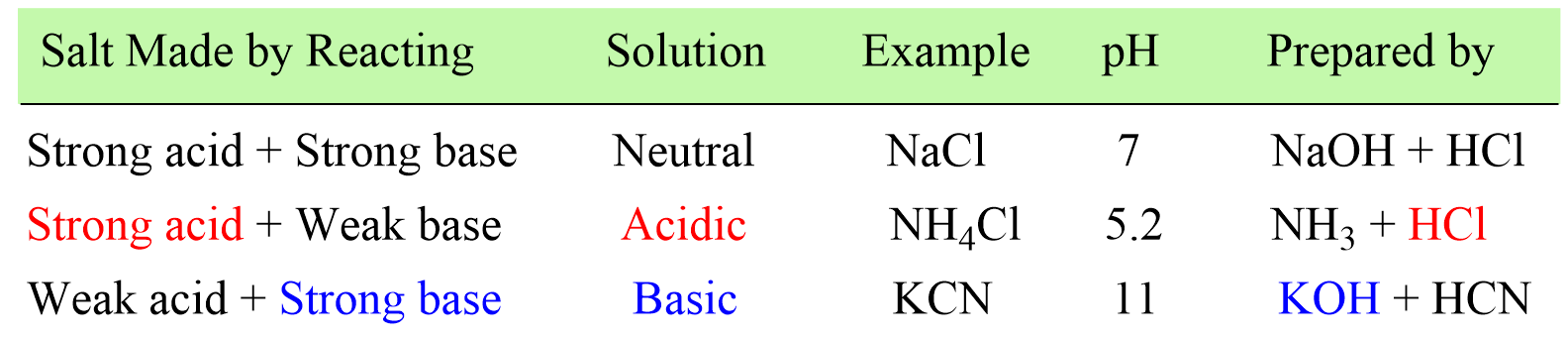(a) KBr is a salt of a strong base and strong acid, and therefore, it is neutral.

(b) FeCl2 is a salt of strong acid HCl, and a weak base Fe(OH)2, and therefore, it is acidic.

(c) Na2CO3 is a salt of a strong base NaOH, and a weak acid H2CO3, and therefore, it is basic.

(d) Al(NO3)3 is a salt of strong acid HNO3, and a weak base Al(OH)3, and therefore, it is acidic.

(e) KClO4 is a salt of a strong base and strong acid, and therefore, it is neutral.

(f) Na2SO3 is a salt of a strong base NaOH, and a weak acid H2SO3, and therefore, it is basic.

(g) NH4ClO3 is a salt of strong acid HClO3, and a weak base NH3, and therefore, it is acidic.

31.

Which of the following salts would produce the most basic aqueous solution?

KF b. NaBr c. NH4Cl d. MgCl2 e. Mg(NO3)2

KF

Solution

NaBr is a salt of a strong base and strong acid, and therefore, it is pH-neutral.

Although Mg(OH)2 is not as strong of a base as NaOH or KOH, it is still considered a strong base. Therefore, MgCl2 and Mg(NO3)2 are neutral because they are prepared from a strong base and a strong acid.

NH4Cl is a salt of strong acid HCl, and a weak base NH3, and therefore, it forms an acidic solution.

KF is a salt of a strong base KOH, and a weak acid HF, and therefore, it forms a basic, or the most basic among the given, solution.

32.

Calculate the pH of a 0.74 M solution of NaOBr (Ka HBrO = 2.90 x 10-9).

pH = 11.2

Solution

NaOBr is a salt of a strong base NaOH and a weak acid HBrO and therefore, the solution will be basic.

To determine the pH of a salt, we dissociate it and write the reaction of its weak component with water. If the reaction produces H+/H3O+, the solution is acidic, and if OH- ions are formed, the solution will be basic.

NaOBr → Na+ + BrO

BrO+ H2O(l) → HBrO(aq)  +  OH(aq)  (basic)

Next, determine the Kb from the Ka:

Ka · Kb = Kw = 10-14

Rearranging this, we get an expression for Kb:

${K_{{\rm{b}}}}\; = \,\frac{{{K_{\rm{w}}}}}{{{K_{\rm{a}}}}}\; = \;\frac{{{\rm{1}}{{\rm{0}}^{{\rm{ – 14}}}}}}{{{\rm{2}}{\rm{.90}}\; \times \;{\rm{1}}{{\rm{0}}^{{\rm{ – 9}}}}}}\; = \;{\rm{3}}{\rm{.45 }} \times {\rm{ 1}}{{\rm{0}}^{{\rm{ – 6}}}}$

Set up an ICE table for Kb assigning x mol/l for ionization.

 [BrO–] [HBrO] [OH–] Initial 0.74 0 0 Change -x +x +x Equil 0.74 – x x x

Write the expression for Kb using the equilibrium concentration sin the ICE table in order to determine [OH].

${K_{{\rm{b}}}}\; = \,\frac{{{\rm{[HBrO][O}}{{\rm{H}}^{\rm{ – }}}{\rm{]}}}}{{{\rm{[Br}}{{\rm{O}}^ – }{\rm{]}}}}\; = \;\frac{{{{\rm{x}}^{\rm{2}}}}}{{{\rm{0}}{\rm{.74}}\;{\rm{ – }}\;{\rm{x}}}}\; \approx \;\frac{{{{\rm{x}}^{\rm{2}}}}}{{{\rm{0}}{\rm{.74}}}} = \;{\rm{3}}{\rm{.4}}\; \times \,{\rm{1}}{{\rm{0}}^{{\rm{ – 6}}}}$

Solving for x, we find that x = 0.0016 mol/l and we check if the approximation was valid:

$\% \, = \;\frac{{0.0016}}{{0.74}}\; \times \;100\% \; = \;0.22\%$

The approximation is valid, so the concentration of OH– ions at equilibrium is 0.0016 M.

We can now calculate the pOH, and then pH using the pH + pH = 14 relationship:

pOH = -log 0.0016 = 2.8, and therefore, the pH is:

pH = 14 – pOH = 14 – 28 = 11.2

And this answer is also reasonable because we predicted to have a basic solution.

33.

Calculate the pH of the 0.26 M solution of NH4ClO3 (Kb NH3 = 1.8 x 10-5).

pH = 4.9

Solution

Ammonium chlorate (NH4ClO3) is the salt of a weak base ammonia (NH3) and a strong acid HClO3, so it yields an acidic solution.

NH3 + HClO3 → NH4ClO3

So, let’s follow what we have discussed earlier and see why it produces an acidic solution.

Dissociate the salt to identify the weak component ion that will react with water:

NH4ClO3 → NH4+ + ClO3

NH4+ is the ion with a weaker component (NH3), so it is going to react with water producing hydronium ion which makes the solution acidic:

NH4+(aq)  + H2O(l) → NH3(aq)  +  H3O+(aq)  (acidic)

The next part is to determine the concentration of H3O+ ions in order to calculate the pH.

We are given the Kb, which measures the base strength and it is based on the reaction of ammonia itself with water:

NH3(aq) + H2O(aq) ⇆ NH4+(aq) + OH(aq)

This equation does not contain H+/H3O+ ions, and we do not know the concentration of ammonia, therefore, we need to determine the Ka.

Ka and Kb can be calculated from one another using the relationship:

Ka · Kb = Kw = 10-14

Rearranging this, we get an expression for Ka:

${K_{\rm{a}}}\; = \,\frac{{{K_{\rm{w}}}}}{{{K_{\rm{b}}}}}\; = \;\frac{{{\rm{1}}{{\rm{0}}^{{\rm{ – 14}}}}}}{{{\rm{1}}{\rm{.8}}\; \times \;{\rm{1}}{{\rm{0}}^{{\rm{ – 5}}}}}}\; = \;{\rm{5}}{\rm{.7 }} \times {\rm{ 1}}{{\rm{0}}^{{\rm{ – 10}}}}$

Once we know the Ka, it is the same procedure as we do when calculating the pH of a weak acid.

We assign x mol/l for ionization and set up an ICE table to determine the [H3O+]:

NH4+(aq)  + H2O(l) → NH3(aq)  +  H3O+(aq)  (acidic)

 [NH4+] [NH3] [H3O+] Initial 0.26 0 0 Change -x +x +x Equil 0.26 – x x x

Next, write the expression for Ka using the equilibrium concentrations in the ICE table:

${K_{{\rm{a}}}}\; = \,\frac{{{\rm{[N}}{{\rm{H}}_{\rm{3}}}{\rm{][}}{{\rm{H}}_{\rm{3}}}{{\rm{O}}^{\rm{ + }}}{\rm{]}}}}{{{\rm{[N}}{{\rm{H}}_{\rm{4}}}^{\rm{ + }}{\rm{]}}}}\; = \;\frac{{{{\rm{x}}^{\rm{2}}}}}{{{\rm{0}}{\rm{.26}}\;{\rm{ – }}\;{\rm{x}}}}\; \approx \;\frac{{{{\rm{x}}^{\rm{2}}}}}{{{\rm{0}}{\rm{.26}}}} = \;{\rm{5}}{\rm{.7 x 1}}{{\rm{0}}^{{\rm{ – 10}}}}$

Therefore, x = 1.2 x 10-5

Check if the approximation was valid:

$\% \, = \;\frac{{1.2\, \times \;{{10}^{ – 5}}}}{{0.26}}\; \times \;100\% \; = \;0.0047\%$

The percentage of hydrolysis is less than 5%, and therefore, the approximation is valid.

The concentration of H3O+ ions is 1.2 x 10-5 M, so the pH is:

pH = -log 1.2 x 10-5 = 4.9

This indicates an acidic solution which is what we expected to have for the solution of NH4ClO3.

34.

Calculate the pH of a 0.35 M solution of KNO2 (Ka = 4.0 x 10-4).

pH = 8.5

Solution

KNO2 is a salt of a strong base KOH and a weak acid HNO2 and therefore, the solution will be basic.

Dissociate the salt and write the reaction of its weak component with water.

KNO2 → K+ + NO2

NO2(aq) + H2O(l) → HNO2(aq) + OH(aq)  (basic)

Next, determine the Kb from the Ka:

Ka · Kb = Kw = 10-14

Rearranging this, we get an expression for Ka:

${K_{{\rm{b}}}}\; = \,\frac{{{K_{\rm{w}}}}}{{{K_{\rm{a}}}}}\; = \;\frac{{{\rm{1}}{{\rm{0}}^{{\rm{ – 14}}}}}}{{{\rm{4}}{\rm{.0}}\; \times \;{\rm{1}}{{\rm{0}}^{{\rm{ – 4}}}}}}\; = \;{\rm{2}}{\rm{.5 }} \times {\rm{ 1}}{{\rm{0}}^{{\rm{ – 11}}}}$

Set up an ICE table for Kb assigning x mol/l for the ionization.

 [NO2–] [HNO2] [OH–] Initial 0.35 0 0 Change -x +x +x Equil 0.35 – x x x

Write the expression for Kb using the equilibrium concentrations in the ICE table in order to determine the [OH].

${K_{{\rm{b}}}}\; = \,\frac{{{\rm{[HN}}{{\rm{O}}_{\rm{2}}}{\rm{][O}}{{\rm{H}}^{\rm{ – }}}{\rm{]}}}}{{{\rm{[N}}{{\rm{O}}_{\rm{2}}}^{\rm{ – }}{\rm{]}}}}\;{\rm{ = }}\;\frac{{{{\rm{x}}^{\rm{2}}}}}{{{\rm{0}}{\rm{.35}}\;{\rm{ – }}\;{\rm{x}}}}\; \approx \;\frac{{{{\rm{x}}^{\rm{2}}}}}{{{\rm{0}}{\rm{.35}}}}{\rm{ = }}\;{\rm{2}}{\rm{.5}}\;{\rm{ \times }}\,{\rm{1}}{{\rm{0}}^{{\rm{ – 11}}}}$

Solving for x, we find that x = 3.0 x 10-6 mol/L, and we check if the approximation was valid:

$\% \, = \;\frac{{3.0\, \times \;{{10}^{ – 6}}}}{{0.35}}\; \times \;100\% \; = \;0.00084\%$

The approximation is valid, so the concentration of OH– ions at equilibrium is 3.0 x 10-6 M.

We can now calculate the pOH, and then pH using the pH + pH = 14 relationship:

pOH = -log 3.0 x 10-6 = 5.5, and therefore, the pH is:

pH = 14 – pOH = 14 – 5.5 = 8.5

And this answer is also reasonable because we predicted a basic solution.

35.

Calculate the pH of a 1.0 M solution of sodium acetate (CH3CO2Na) considering that the Ka of acetic acid (CH3CO2H is 1.7 x 10-5.

pH = 9.4

Solution

CH3CO2Na is a salt of a strong base NaOH and a weak acid CH3CO2H therefore, the solution will be basic.

Dissociate the salt and write the reaction of its weak component with water.

CH3CO2Na → Na+ + CH3CO2

CH3CO2(aq) + H2O(l) → CH3CO2H(aq) + OH(aq)  (basic)

Next, determine the Kb from the Ka:

Ka · Kb = Kw = 10-14

Rearranging this, we get an expression for Ka:

${K_{{\rm{b}}}}\; = \,\frac{{{K_{\rm{w}}}}}{{{K_{\rm{a}}}}}\; = \;\frac{{{\rm{1}}{{\rm{0}}^{{\rm{ – 14}}}}}}{{{\rm{1}}{\rm{.7}}\; \times \;{\rm{1}}{{\rm{0}}^{{\rm{ – 5}}}}}}\; = \;{\rm{5}}{\rm{.9 }} \times {\rm{ 1}}{{\rm{0}}^{{\rm{ – 10}}}}$

Set up an ICE table for Kb assigning x mol/l for the ionization.

CH3CO2(aq) + H2O(l) → CH3CO2H(aq) + OH(aq)

 [CH3CO2–] [CH3CO2H] [OH–] Initial 1.0 0 0 Change -x +x +x Equil 1.0 – x x x

Write the expression for Kb using the equilibrium concentrations in the ICE table in order to determine [OH].

${K_{{\rm{b}}}}\; = \,\frac{{{\rm{[C}}{{\rm{H}}_{\rm{3}}}{\rm{C}}{{\rm{O}}_{\rm{2}}}{\rm{H][O}}{{\rm{H}}^{\rm{ – }}}{\rm{]}}}}{{{\rm{[C}}{{\rm{H}}_{\rm{3}}}{\rm{C}}{{\rm{O}}_{\rm{2}}}^{\rm{ – }}{\rm{]}}}}\;{\rm{ = }}\;\frac{{{{\rm{x}}^{\rm{2}}}}}{{{\rm{1}}{\rm{.0}}\;{\rm{ – }}\;{\rm{x}}}}\; \approx \;\frac{{{{\rm{x}}^{\rm{2}}}}}{{{\rm{1}}{\rm{.0}}}}{\rm{ = }}\;{\rm{5}}{\rm{.9}}\;{\rm{ \times }}\,{\rm{1}}{{\rm{0}}^{{\rm{ – 10}}}}$

We find that x = 2.4 x 10-5 mol/l.

Check if the approximation was valid:

$\% \, = \;\frac{{2.4\, \times \;{{10}^{ – 5}}}}{{1.0}}\; \times \;100\% \; = \;0.0024\%$

The approximation is valid, so the concentration of OH– ions at equilibrium is 3.0 x 10-6 M.

We can now calculate the pOH, and then pH using the pH + pH = 14 relationship:

pOH = -log 2.4 x 10-5 = 4.6, and therefore, the pH is:

pH = 14 – pOH = 14 – 4.6 = 9.4

And this answer is also reasonable because we predicted to have a basic solution.

1686021606